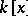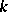# Algebraically closed field

(diff) ← Older revision | Latest revision (diff) | Newer revision → (diff)
A fieldin which any polynomial of non-zero degree overhas at least one root. In fact, it follows that for an algebraically closed fieldeach polynomial of degreeoverhas exactlyroots in, i.e. each irreducible polynomial from the ring of polynomialsis of degree one. A fieldis algebraically closed if and only if it has no proper algebraic extension (cf. Extension of a field). For any field, there exists a unique (up to isomorphism) algebraic extension ofthat is algebraically closed; it is called the algebraic closure ofand is usually denoted by. Any algebraically closed field containingcontains a subfield isomorphic to.